基于简化Lorenz混沌电路的频率特性分析Frequency Characteristics Analysis Based on Simplified Lorenz System

• 全文下载: PDF(1549KB)    PP.93-100   DOI: 10.12677/OJCS.2018.74012
• 下载量: 175  浏览量: 275   科研立项经费支持

In the hardware circuit of the chaotic system, the integral constant of the integrating circuits can be configured to change the size of the system time scale transformation. However, the different parameter configurations of the integrating circuits will change the frequency distribution range of the system signal. What effect does the change in the signal frequency distribution have on the nonlinear dynamic characteristics of the system? In this paper, the variation of the oscillation frequency range of the system signal is observed by changing the integral time constant in chaotic circuit. The circuit simulation of the simplified Lorenz system is used to analyze the oscillation frequency range and explore the potential influence for the chaotic system with different parameter configuration of the integrating circuits. The experimental results have showed that the different parameter configuration of integrating circuits can change the oscillation frequency range of the chaotic system signal, but it does not change the chaotic characteristics of the system. In the chaotic systems based on memristors whose volt-ampere characteristics exhibited vibration with frequency, the parameter configuration of integrating circuits might cause potential effect on nonlinear dynamic characteristics of the system.
<>

1. 引言

2. 简化Lorenz系统

$\left\{\begin{array}{l}\stackrel{˙}{x}=10\left(y-x\right)\\ \stackrel{˙}{y}=\left(24-4c\right)x-xz+cy\\ \stackrel{˙}{z}=xy-8z/3\end{array}$ (1)

$\left\{\begin{array}{l}\text{d}x/\text{d}t=\frac{1}{{R}_{5}{C}_{1}}\left(\frac{{R}_{3}}{{R}_{1}}y-\frac{{R}_{4}\left({R}_{1}+{R}_{3}\right)}{{R}_{1}\left({R}_{2}+{R}_{4}\right)}x\right)\\ \text{d}y/\text{d}t=\frac{1}{{R}_{17}{C}_{2}}\left(\frac{{\text{R}}_{16}}{{R}_{8}}x-\frac{{R}_{16}{R}_{7}}{10{R}_{9}{R}_{6}}xz+\frac{{R}_{16}{R}_{14}}{{R}_{15}}\left(\frac{{R}_{11}}{{R}_{12}{R}_{10}}y-\frac{1}{{R}_{13}}x\right)\right)\\ \text{d}z/\text{d}t=\frac{1}{{R}_{22}{C}_{3}}\left(\frac{{R}_{20}}{10{R}_{18}}xy-\frac{{R}_{21}\left({R}_{18}+{R}_{20}\right)}{{R}_{18}\left({R}_{21}+{R}_{19}\right)}z\right)\end{array}$ (2)Figure 1. Phase portraits of chaotic attractor (x-y plane, x-z plane)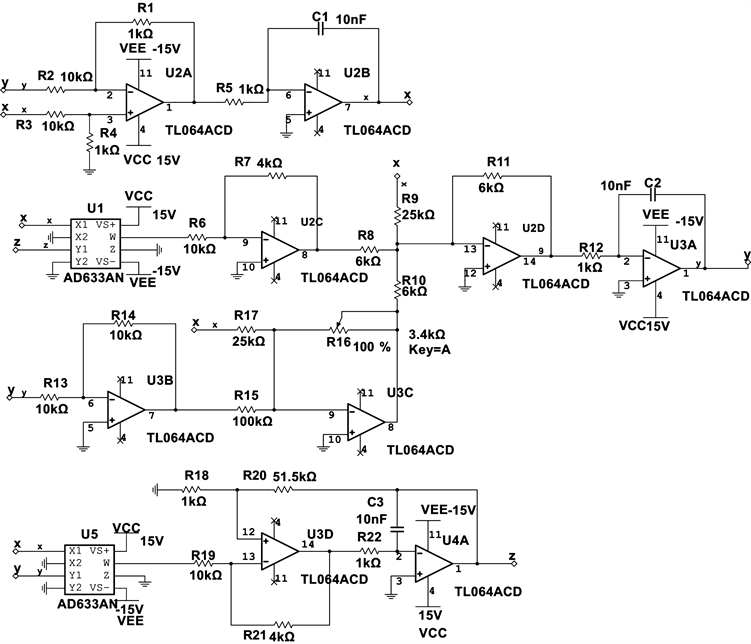Figure 2. Circuit of simplified Lorenz system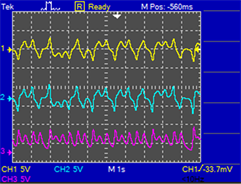Figure 3. The signals x(t), y(t), z(t) of simplified Lorenz circuit (R = 1 kΩ, R14 = 3.4 kΩ). (a) C = 1 nF; (b) C = 100 nF; (c) C = 4.7 uF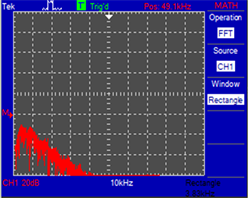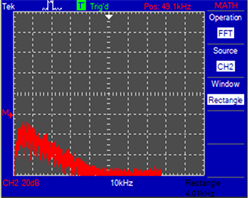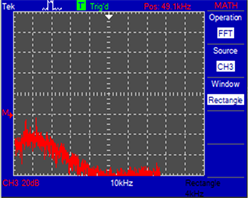(a)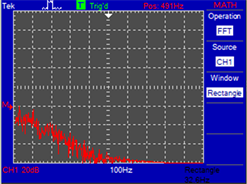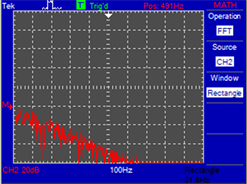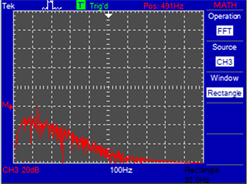(b)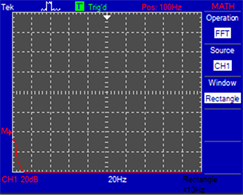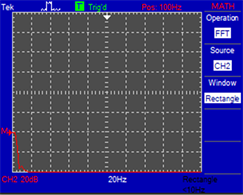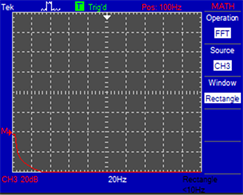(c)

Figure 4. Spectrum of signals x(t), y(t), z(t) of simplified Lorenz circuit (R = 1 kΩ, R14 = 3.4 kΩ). (a) C = 1 nF; (b) C = 100 nF; (c) C = 4.7 uF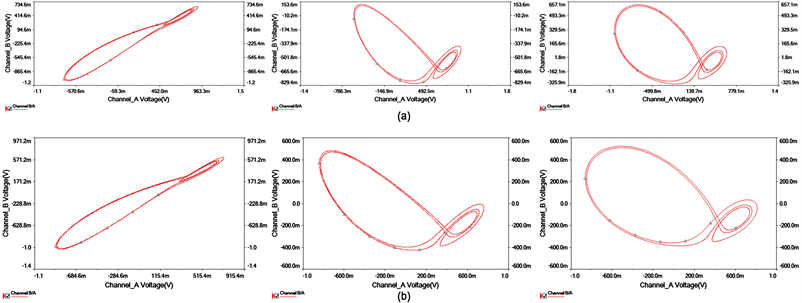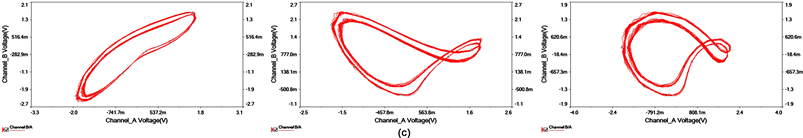Figure 5. Phase portraits of attractors with different parameters. (a) R14 = 7.38 kΩ, C = 4.7 uF; (b) R14 = 7.38 kΩ, C = 100 nF; (c) R14 = 5.55 kΩ, C = 1 nF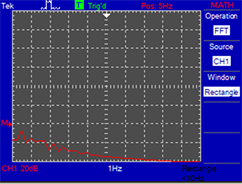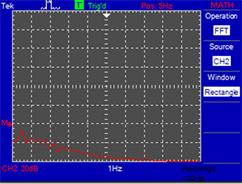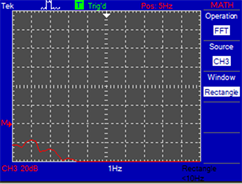(a)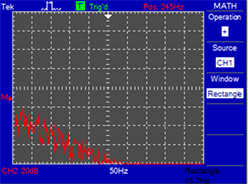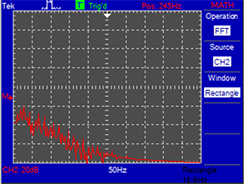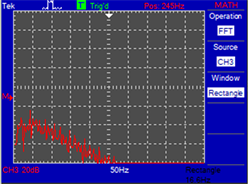(b)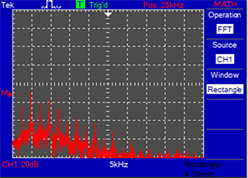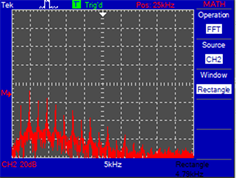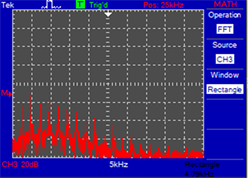(c)

Figure 6. The spectrogram of x(t), y(t), z(t) in non-chaotic state. (a) R14 = 7.38 kΩ, C = 4.7 uF; (b) R14 = 7.38 kΩ, C = 100 nF; (c) R14 = 5.55 kΩ, C = 1 nF

3. 结论

NOTES

*通讯作者。

  Strukov, D.B., Snider, G.S., Stewart, D.R., et al. (2008) The Missing Memristor Found. Nature, 453, 80-83. https://doi.org/10.1038/nature06932  Wang, L., Drakakis, E., Duan, S., et al. (2012) Memristor Model and Its Application for Chaos Generation. International Journal of Bifurcation and Chaos, 22, 241-252. https://doi.org/10.1142/S0218127412502057  欧青立, 徐林波, 郭子叶, 等. 基于忆阻器的五阶MCK混沌电路研究[J]. 量子电子学报, 2016, 33(1): 56-62.  李志军, 曾以成. 基于文氏振荡器的忆阻混沌电路[J]. 电子与信息学报, 2014, 36(1): 88-93.  谭志平, 曾以成, 李志军, 等. 浮地型忆阻器混沌电路的分析与实现[J]. 仪器仪表学报, 2014, 35(9): 2123-2129.  王伟, 曾以成, 孙睿婷. 含三个忆阻器的六阶混沌电路研究[J]. 物理学报, 2017, 66(4): 25-35.  阮静雅, 孙克辉, 牟俊. 基于忆阻器反馈的Lorenz超混沌系统及其电路实现?[J]. 物理学报, 2016, 65(19): 19-29.  Bao, B.C., Xu, J.P., Liu, Z., et al. (2011) Dynamics Analysis of Chaotic Circuit with Two Memristors. Science China Technological Sciences, 54, 2180-2187. https://doi.org/10.1007/s11431-011-4400-6  Yan, W., Sun, K., He, S., et al. (2014) Dynamics of Fraction-al-Order Sinusoidally Forced Simplified Lorenz System and Its Synchronization. European Physical Journal Special Topics, 223, 1591-1600. https://doi.org/10.1140/epjst/e2014-02181-3  孙克辉, 杨静利, 丁家峰, 等. 单参数Lorenz混沌系统的电路设计与实现[J]. 物理学报, 2010, 59(12): 8385-8392.  Sun, K., Liu, X., Zhu, C., et al. (2012) Hyperchaos and Hyperchaos Control of the Sinusoidally Forced Simplified Lorenz System. Nonlinear Dynamics, 69, 1383-1391. https://doi.org/10.1007/s11071-012-0354-x  Wang, X., Chen, J., Lu, J., et al. (2012) A Simple Yet Complex Oneparameter Family of Generalized Lorenz-Like Systems. International Journal of Bifurcation and Chaos, 22, 1250116-1250116. https://doi.org/10.1142/S0218127412501167  孙克辉. 混沌保密通信原理与技术[M]. 北京: 清华大学出版社, 2015: 49-52.  Valsa, J., Biolek, D. and Biolek, Z. (2011) An Analogue Model of the Memristor. International Journal of Numerical Modelling Electronic Networks Devices & Fields, 24, 400-408. https://doi.org/10.1002/jnm.786  王晓媛, 齐维贵, 王兴元. 忆阻器的电路实现及其混沌动力学研究[J]. 北京航空航天大学学报, 2012, 38(8): 1080-1084.  Bao, B.C., Xu, J.P., Zhou, G.H., et al. (2011) Chaotic Memristive Circuit: Equivalent Circuit Realization and Dynamical Analysis. Chinese Physics B, 20, 109-115.